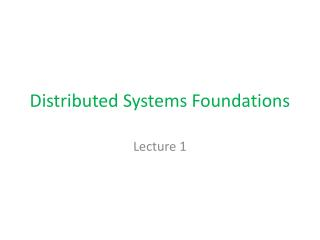# Distributed Systems Foundations - PowerPoint PPT PresentationDownload PresentationDistributed Systems Foundations

Distributed Systems Foundations
Download Presentation## Distributed Systems Foundations

- - - - - - - - - - - - - - - - - - - - - - - - - - - E N D - - - - - - - - - - - - - - - - - - - - - - - - - - -
##### Presentation Transcript

1. Distributed Systems Foundations Lecture 1

2. Main Characteristics of Distributed Systems • Independent processors, sites, processes • Message passing • No shared memory • No shared clock • Independent failure modes CS 271

3. Distributed System Models • Synchronous System: Known bounds on times for message transmission, processing , bounds on local clock drifts, etc. • Can use timeouts • AsynchronousSystem: No known bounds on times for message transmission, processing, bounds on local clock drifts, etc. • More realistic, practical, but no timeout. CS 271

4. Causality and Time CS 271

5. What is a Distributed System? • A simple model of a distributed system proposed by Lamport in a landmark 1978 paper: • “Time, Clocks and the Ordering of Events in a Distributed System” Communications of the ACM CS 271

6. What is a Distributed System? • A set of processes that communicate using message passing. • A process is a sequence of events • 3 kinds of events: • Local events • Send events • Receive events • Local events on a process for a total order. CS 271

7. Happens Before or Causal Order on Events • Event e happens before (causally precedes)event f, denoted e → f if: • The same process executes e before f ; or • e is send(m)and f is receive(m);or • Existshso thate → h and h → f • We define concurrent, e || f, as: ¬(e → f f → e) CS 271

8. Lamport Logical Clocks • Assign “clock” value to each event such that • if ab then clock(a) < clock(b) • Assign each process a clock “counter”. • Clock must be incremented between any two events in the same process • Each message carries the sender’s clock value • When a message arrives set local clock to: • max(local value, message timestamp + 1) • This clock forms a partial order. If a total order is needed use process ids to break ties—totally ordered Lamport (or Logical) Clocks CS 271

9. Vector clocks • Vector initialized to 0 at each processVi [j] = 0 for i, j =1, …, N • Process increments its element of the vector in local vector before event:Vi [i] = Vi [i] +1 • Piggyback Vi with every message sent from process Pi • When Pj receives message, compares vectors element by element and sets local vector to higher of two values Vj[k] = max(Vj[k], Vi[k]) for k=1, …, N CS 271

10. Comparing vector timestamps Define V = V’iffV[i] = V’[i] for i= 1 … NV  V’iffV[i]  V’[i] for i= 1 … N For any two events e, e’ e  e’ if and only if V(e) < V(e’) Two events are concurrent if neither V(e)  V(e’) nor V(e’)  V(e) CS 271

11. Vector Clock Example CS 271

12. Clock Synchronization • Assume each process p has a physical clock Cp. • Assume an accurate time source providing Universal Coordinated Time (UCT)—atomic clock or GPS. • Clock Synchronization: • Keep all clocks in a distributed system close to each other, ie, synchronized. • If UCT is t, and value of clock at process p is Cp(t) then ideally, Cp(t) = t, ie, dCp(t)/dt = 1. CS 271

13. Clock Synchronization • The internal timer causes an interrupt h times a second. • When interrupt occurs, clock is incremented, and clock keeps times since it was initialized. • In reality, timers have drift ρ therefore: 1-ρ =< dCp(t)/dt =< 1+ ρ • Two clocks can drift 2 ρΔt afterΔt. • If we want clocks not to differ by more than δ, then resynch at least every δ/2ρsecs

14. Cristian’s Clock Synch Algorithm [Distributed Computing 1989] • A processor p requests time from UTC. • UTC sends back current time t. • What does p set local clock to? t + Tround/2 • Tround = treceive –tsend. • Assume minimum time is min. • Error: +/- [Tround/2 –min] • Time must be monotonic. What if t is less than current time at p? CS 271